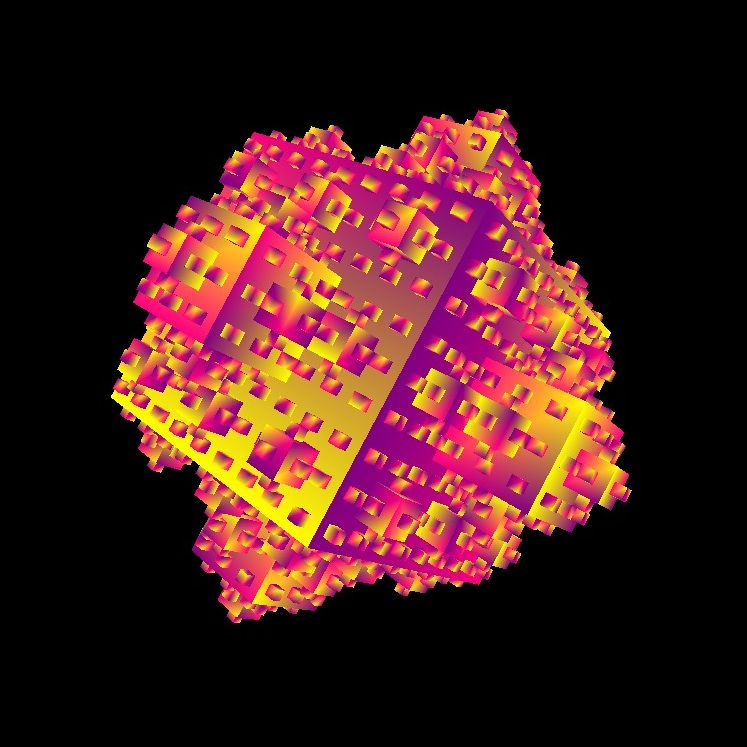Last edited 19dec13 by semibra2@illinois.edu

Find this document at http://new.math.uiuc.edu/math198/MA198-2013/semibra2/

Higher-Dimensional Koch Curves

Yuliya SemibratovaAbstract:

The optimization of materials is a key part of materials science and engineering. Interestingly, the perfecting process often takes advantage of the natural problems present in materials, entitled defects. Typically, these defects are classified according Euclidean dimensions (0, 1, 2, etc.). While this approach works for certain defects, such as those in Table 1.1: Euclidean Defects, it does not work for defects that are curvilinear or those that cannot be described using standard equations.

The other defects, since they do not exist in any Euclidean (integer) dimension, must lie in fractional (fractal) dimension, denoted by D (see Table 1.1: Types of Defects). One such defect, the grain boundary, whose dimension is between one and two, can be used to measure the fracture toughness of the material. such, production processes that vary the fractal dimension of the grain boundary can be used to strengthen or weaken a material.

According to studies by H. Khanbareh, J. H. Kruhl, and M. Nega, grain boundaries can be approximated by the Koch Curve fractal. The traditional Koch curve, which starts with a one-dimensional line segment and exists in the dimension log4/log3, repeats according to the following process:

1. Take an equilateral triangle.
2. Split the sides into three equal lengths.
3. On any outward facing side (a side that is not connected to a previous triangle), take the central length and create an equilateral triangle from it.
4. For each line segment in the set, repeat from 1.

Other versions of the Koch curve manipulate the initial line segment in different manners. Technically, the curve will remain a Koch curve fractal if it is self-similar and exists in a fractional dimension between one and two.

With some extrapolation, the Koch process can be extended to higher dimensional starting points. In particular, if the starting point is a three-dimensional object, such as a regular tetrahedron or a cube (analogs of the triangular and square patterns for 1<D<2 Koch curves), then the resulting form will be a fractal with 2<D<3.

Table 1.1: Types of Defects

 Zero-dimensional Point defect Vacancy Interstitial Substitutional One-dimensional Line defect Dislocation Two-dimensional Plane defect Twin planes Three-dimensional Three-dimensional defect Fractal dimension Grain boundaries (1